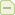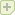flash 贪吃蛇游戏的制作

Flash游戏教程 2006-8-24 10:17

Flash 动画

贪吃蛇游戏，因为制作简单，逻辑性较强，通常是学做游戏的必练的项目。很久以前，我曾经

flash 中进行游戏设计时，界面的可视操作，是其最大的优点，利用它自身的优点，可以将精

在flash中设计时需要考虑的问题主要是键盘控制，mc的加载与御载，一些变量的初始化，以及

在本次设计中，规划了三个主页面的结构，第一个界面用于显示封面，第二个是游戏界面，第

为了实现参数化的效果，这里使用了一个boundary_mc 用来表示可运动的范围，系统中一切的

var init_length = 2;

var dir = 1;

var speed = 15;

在游戏设计中，数据结构是一个需要首先考虑的问题，flash中已经可以摆脱类似链表的复杂结

、身体(body)、尾巴(tail)。代码如下：

// ============== create snake ===========

var mov_array:Array = new Array();

head._x = boundary_mc._x + head._width / 2;

head._y = boundary_mc._y + boundary_mc._height - head._height / 2;

mov_array.push(body);

body._visible = false;

for (var i = 0; i < init_length; i++) {

duplicateMovieClip(body, "body" + i, i);

mov_array.push(_root["body" + i]);

_root["body" + i]._x = head._x;

_root["body" + i]._y = head._y;

//trace(mov_array[i]._x);

}

mov_array.push(tail);

function s_move() {

for (var i = mov_array.length - 1; i > 0; i--) {

mov_array[i]._x = mov_array[i - 1]._x;

mov_array[i]._y = mov_array[i - 1]._y;

}

}

// ============ create food ===========

function create_food() {

food_mc._x = Math.floor(Math.random() * 19) * head._width + boundary_mc._x + 8;

food_mc._y = Math.floor(Math.random() * 15) * head._height + boundary_mc._y + 8;

}

food_interval = setInterval(create_food, 5000);

function eat_food() {

var i = mov_array.length - 2;

duplicateMovieClip(body, "body" + i, i);

mov_array.pop();

mov_array.push(_root["body" + i]);

mov_array.push(tail);

create_food();

clearInterval(food_interval);

food_interval = setInterval(create_food, 5000);

}

}

function check_rulesI() {

var left_x = boundary_mc._x;

var right_x = boundary_mc._x + boundary_mc._width;

var top_y = boundary_mc._y;

var button_y = boundary_mc._y + boundary_mc._height;

if (head._x < left_x || head._x > right_x || head._y < top_y || head._y > button_y) {

return true;

} else {

return false;

}

}

function check_rulesII() {

var left_x = boundary_mc._x;

var right_x = boundary_mc._x + boundary_mc._width;

var top_y = boundary_mc._y;

var button_y = boundary_mc._y + boundary_mc._height;

if (head._x < left_x) {

head._x = right_x - head._width / 2;

}

if (head._x > right_x) {

head._x = left_x + head._width / 2;

}

if (head._y < top_y) {

head._y = button_y - head._height / 2;

}

if (head._y > button_y) {

head._y = top_y + head._height / 2;

}

if (mov_array.length > 22) {

return true;

} else {

return false;

}

}

，比如时间未到食物就跑开了，因为多个定时器同时起作用了。在设定定时器时使用的名称

food_interval，只是对定时器的引用，直接再给它设一个定时器，则前面设定的定时器仍存在，所

function end_game() {

clearInterval(food_interval);

//弹出尾部

mov_array.pop();

while (mov_array.length > 1) {

//逐个弹出并删除身体。

removeMovieClip(mov_array[mov_array.length - 1]);

mov_array.pop();

}

Key.removeListener(keyListener);

gotoAndStop("game over");

}

onEnterFrame = function () {

switch (dir) {

case 1 :

break;

case 2 :

break;

case 3 :

break;

case 4 :

break;

}

if (check_rulesII()) {

delete this.onEnterFrame;

end_game();

}

s_move();

eat_food();

};

var keyListener:Object = new Object();

keyListener.onKeyDown = function() {

if (Key.getCode() == Key.RIGHT) {

dir = 1;

} else if (Key.getCode() == Key.DOWN) {

dir = 2;

} else if (Key.getCode() == Key.LEFT) {

dir = 3;

} else if (Key.getCode() == Key.UP) {

dir = 4;

}

};

(提示：蛇的头、身、尾是和代码不相关的，所以可以设计一个更炫的图形来表示一下，留给你自己

////作者与2007年6月26日补充：

《贪吃蛇的制做》已经写成完整的教程并发表在博客中，详情请参阅下面这篇文章：

blog.5d.cn/user12/dzxz/200611/330066.html 内附原代码下载。{commentauthor}
{commenttime}
{commentnum}
{commentcontent}
作者:
{commentrecontent}
﻿

注册5D通行证,立刻拥有自己的博客Join 5D!发表留言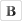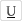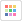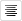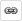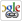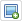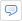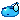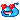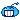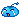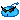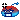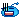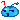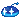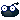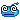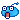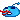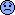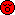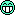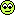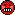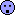禁止笑脸转换 No smiley